﻿ 基于HyPneu的混凝土泵车臂架液压系统仿真分析 Simulation Analysis Based on HyPneu for Concrete Pump Truck’s Arms

International Journal of Fluid Dynamics
Vol. 06  No. 04 ( 2018 ), Article ID: 27540 , 7 pages
10.12677/IJFD.2018.64010

Simulation Analysis Based on HyPneu for Concrete Pump Truck’s Arms

Xiao Lei, Jiaxiang Lin*, Zhuo Liu, Xiaoqi Yang

School of Mechanical Engineering, Guangxi University of Science and Technology, Liuzhou Guangxi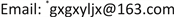Received: Oct. 23rd, 2018; accepted: Nov. 7th, 2018; published: Nov. 14th, 2018ABSTRACT

We present the development of the concrete pump truck, and introduce the fluid simulation software HyPneu function. We analyze the working principle of the hydraulic system of the concrete pump truck’s arm, using HyPneu software to build the schematic diagram of the hydraulic system, simulating the stretching process of the concrete pump truck’s arm system, obtaining the pressure and displacement curve of each arm cylinder. At the same time, we optimize the size of each arm cylinder with HyPneu, and the optimized size can reduce the full stroke time of each arm cylinder. The simulation results agree well with the experimental data, verifying the rationality of hydraulic system design. The simulation results are helpful to the design of the hydraulic system of the concrete pump truck’s arm.

Keywords:Concrete Pump Truck, Arm, Hydraulic System, HyPneu, Simulation1. 引言

HyPneu软件是美国BarDyne公司开发的一款集液压、气动分析为一体的流体动力与运动控制设计仿真与过程可视化的软件  。软件包含了前、后处理、仿真计算与动画演示功能，可为工程设计人员提供了分析和解决液压、气动领域问题的CAE手段，并提供了对工程验证、改型设计、新产品研发的辅助支持，以及作为液压、气动、机械、电子、电磁一体化系统分析的虚拟仿真平台，实现多学科多领域的联合仿真。本研究利用HyPneu软件对混凝土泵车臂架液压系统进行仿真分析，获取臂架获取臂架液压缸伸展过程的动态参数，验证合理性并进行优化。

2. 混凝土臂架液压系统原理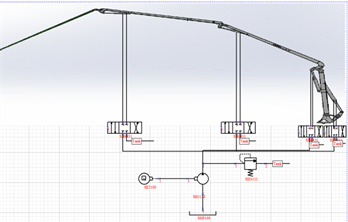Figure 1. Hydraulic system Hypneu schematic of the arm frame

3. 液压系统仿真

3.1. 液压系统建模1.油箱，2.液压泵，3.电机，4.溢流阀，5.三位四通换向阀，6.三位四通换向阀，7.三位四通换向阀，8.三位四通换向阀，9.信号元件，10.信号元件，11.信号元件，12.信号元件，13.第一节臂架液压缸，14.第二节臂架液压缸，15.第三节臂架液压缸，16.第四节臂架液压缸，17.负载等效模型

Figure 2. Hydraulic simulation system of the arm frame

3.2. 液压泵建模

${\eta }_{VP}={A}_{0}+{A}_{1}\Delta P$ (1)Table 1. Volumetric efficiency of the pump under different pressure conditions

${\eta }_{VP}=-0.0002\Delta P+1$ (2)

3.3. 负载建模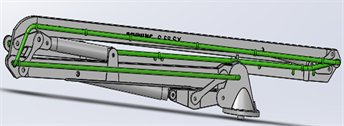Figure 3. Simulation model of the arm frame

$F\left(x\right)=0.0808{x}^{4}-14.49{x}^{3}+889.16{x}^{2}-19745x+145341$ (第一节臂架液压缸) (3)

$F\left(x\right)=-0.0002{x}^{4}-0.1556{x}^{3}+22.623{x}^{2}+187.15x-446$ (第二节臂架液压缸) (4)

$F\left(x\right)=-2.6862{x}^{3}+332.64{x}^{2}-10571x+109779$ (第三节臂架液压缸) (5)

$F\left(x\right)=0.0502{x}^{4}-8.6468{x}^{3}+551.64{x}^{2}-14474x+135939$ (第四节臂架液压缸) (6)

$0\text{cm}\le x\le 5.6\text{cm}$

4. 液压系统仿真分析

4.1. 仿真结果分析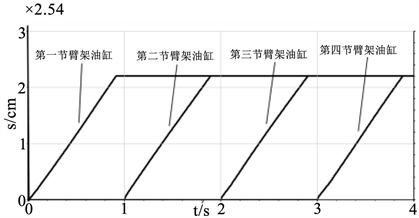Figure 4. Displacement curve of the arm hydraulic cylinder

4.2. 液压系统优化设计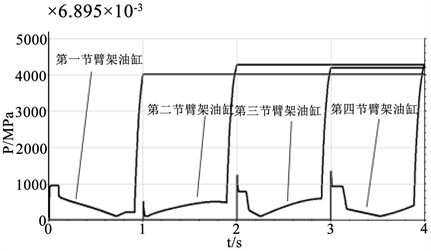Figure 5. Pressure curve of the arm hydraulic cylinder without rod cavity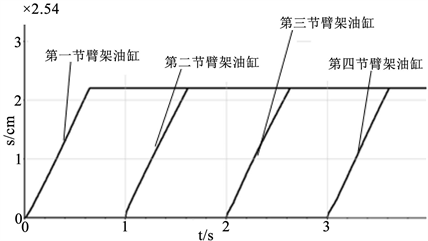Figure 6. Displacement curve of the arm cylinder

5. 结论

Simulation Analysis Based on HyPneu for Concrete Pump Truck’s Arms[J]. 流体动力学, 2018, 06(04): 78-84. https://doi.org/10.12677/IJFD.2018.64010

1. 1. 刘会勇. 混凝土泵车臂架液压系统建模与仿真研究[J]. 工程设计学报, 2010, 17(4): 1-2.

2. 2. 张仕全. 混凝土泵车臂架液压系统动态建模与仿真[D]: [硕士学位论文]. 长沙: 长沙理工大学, 2004: 50-60.

3. 3. 王普茂. 机械制造业中液压技术的应用及前景分析[J]. 液压与气动, 2012(4): 111-113.

4. 4. 丘铭军, 郭星良, 艾春璇, 等. 结晶器在线热调宽液压伺服系统仿真与试验研究[J]. 重型机械, 2012(5): 3-4.

5. 5. 冯永仁, 秦小飞, 潘明峰. 基于HyPneu的地层测试器推靠坐封液压系统仿真分析[J]. 液压与气动, 2014(6): 24-28, 50.

6. 6. 李昆. 基于HyPneu的某型机襟翼系统建模与仿真计算[J]. 飞机工程, 2006(4): 1-2.

7. 7. 李昆. 某型飞机纵向飞控–液压系统协同仿真技术研究[C]. 第五届全国流体传动与控制学术会议暨2008年中国航空学会液压与气动学术会议, 2008: 3.

8. 8. 刘其伟. 50 t混凝土试验台液压加载系统设计与仿真研究[D]: [硕士学位论文]. 安徽: 安徽工业大学, 2016: 16-40.

9. NOTES

*通讯作者。